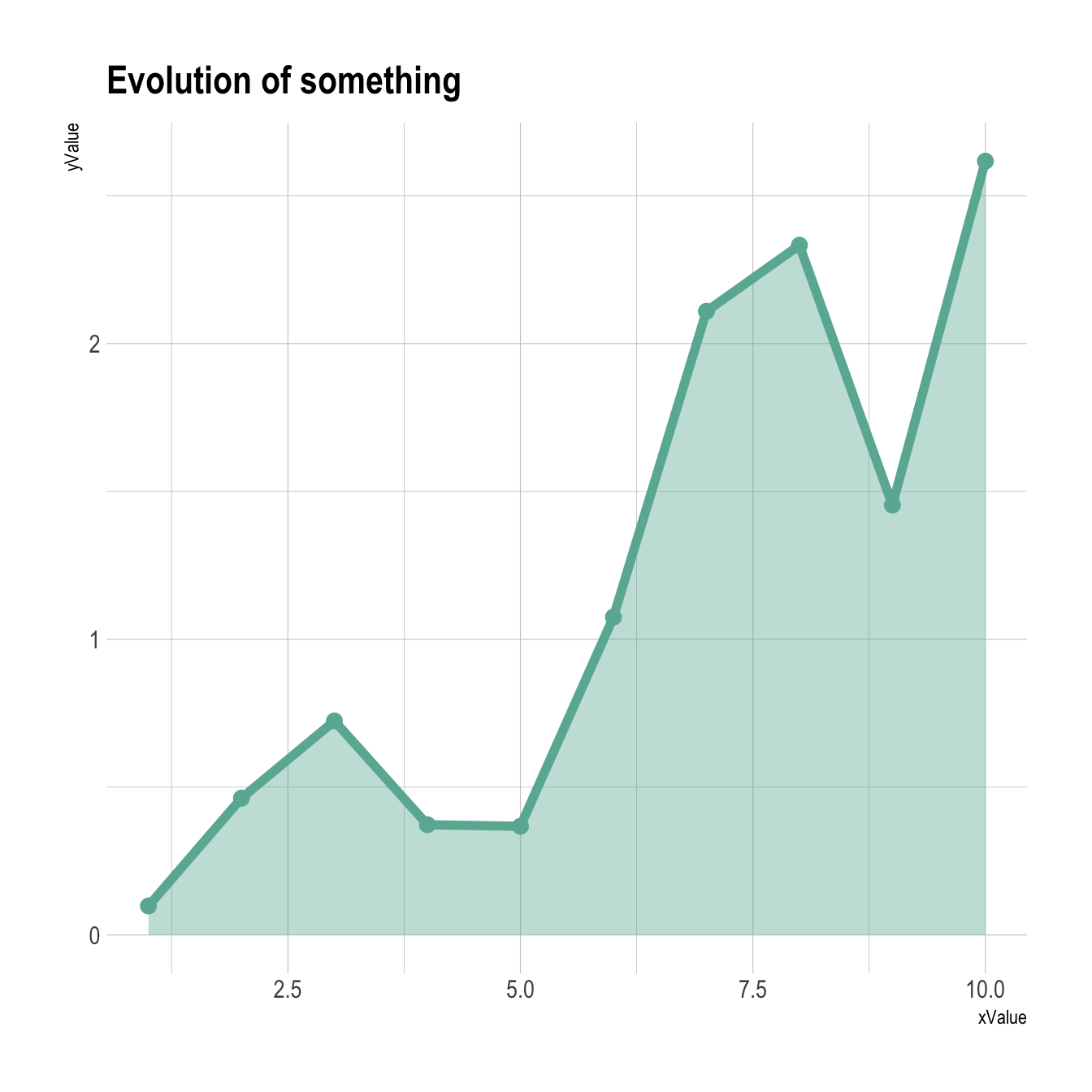# Area chart with R and ggplot2

This post is a step by step introduction to area chart with R and ggplot2. It provides several reproducible examples with explanation and R code.

# Basic line chart with `ggplot2` and `geom_line()`

An area chart displays the evolution of one or several numeric variables. Data points are usually connected by straight line segments, and the area between the X axis and the line is filled. See data-to-viz for a more in-depth definition.

As for a line chart, the input data frame requires at least 2 columns:

• An ordered numeric variable for the X axis
• Another numeric variable for the Y axis

Once the data is read by ggplot2 and those 2 variables are specified in the `x` and `y` arguments of the `aes()`, just call the `geom_area()` function.``````# Libraries
library(ggplot2)

# create data
xValue <- 1:50
yValue <- cumsum(rnorm(50))
data <- data.frame(xValue,yValue)

# Plot
ggplot(data, aes(x=xValue, y=yValue)) +
geom_area()``````

# Customize the line chart

Several options are available to customize the area chart appearance:

• Add a title with `ggtitle()`.
• Change line style with arguments like `shape`, `size`, `color` and more.
• Add transparency to the filled region with `opacity`
• Custom the general theme with the `theme_ipsum()` function of the `hrbrthemes` package.
• Highlight the top line with `geom_line()`
• If not too many observation, show individual data points with `geom_point()`

More generally, visit the [ggplot2 section] for more ggplot2 related stuff.``````# Libraries
library(ggplot2)
library(hrbrthemes)

# create data
xValue <- 1:10
yValue <- abs(cumsum(rnorm(10)))
data <- data.frame(xValue,yValue)

# Plot
ggplot(data, aes(x=xValue, y=yValue)) +
geom_area( fill="#69b3a2", alpha=0.4) +
geom_line(color="#69b3a2", size=2) +
geom_point(size=3, color="#69b3a2") +
theme_ipsum() +
ggtitle("Evolution of something")``````

Related chart types

## Contact

This document is a work by Yan Holtz. Any feedback is highly encouraged. You can fill an issue on Github, drop me a message on Twitter, or send an email pasting yan.holtz.data with gmail.com.